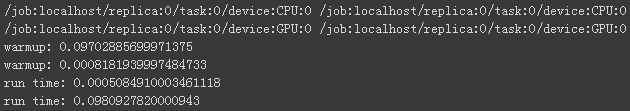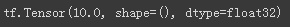# Tensorflow2--功能演示

2020年04月11日 12点热度 0人点赞 0条评论

深度学习的核心是算法的设计思想，深度学习框架只是我们实现算法的工具。下面我们将演示 TensorFlow 深度学习框架的 3 大核心功能，从而帮助我们理解框架在算法设计中扮演的角色。

### a) 加速计算

'''

1.TensorFlow 的重要功能就是利用 GPU 方便地实现并行计算加速功能。

'''
import tensorflow as tf
import timeit
# import keras.backend as K
# cfg = K.tf.ConfigProto(gpu_options={'allow_growth': True})
# K.set_session(K.tf.Session(config=cfg))
n=10000000
#创建在CPU上运算的2个矩阵
with tf.device('/cpu:0'):
cpu_a=tf.random.normal([1,n])#从正态分布生成随机值
cpu_b=tf.random.normal([n,1])
#print(cpu_a,'n',cpu_b)
print(cpu_a.device,cpu_b.device)

#创建使用GPU运算的2个矩阵
with tf.device('/gpu:0'):
gpu_a=tf.random.normal([1,n])
gpu_b=tf.random.normal([n,1])
print(gpu_a.device,gpu_b.device)
#并通过time.timeit()函数来测量2个矩阵的运算时间：
def cpu_run():
with tf.device('/cpu:0'):
c = tf.matmul(cpu_a,cpu_b)
return c

def gpu_run():
with tf.device('/gpu:0'):
c = tf.matmul(gpu_a,gpu_b)
return c

#第一次计算需要热身，避免将初始化阶段时间结算在内
cpu_time = timeit.timeit(cpu_run,number=10)
print('warmup:',cpu_time)
gpu_time = timeit.timeit(gpu_run,number=10)
print('warmup:',gpu_time)

#正式计算10次，取平均时间
cpu_time = timeit.timeit(cpu_run,number=10)
gpu_time = timeit.timeit(gpu_run,number=10)
print('run time:',gpu_time)
print('run time:',cpu_time)### b) 自动梯度

通过 TensorFlow 实现如下

import tensorflow as tf

#创建4个张量
a = tf.constant(1.)
b = tf.constant(2.)
c = tf.constant(3.)
w = tf.constant(4.)

tape.watch([w]) #将w加入梯度跟踪队列
#构建计算过程
y = a*w**2+b*w+c

#求导
print(dy_dw) #打印出导数### c) 常用神经网络接口

TensorFlow 除了提供底层的矩阵相乘，相加等运算函数，还内建了常用网络运算函数，常用网络层，网络训练，网络保存与加载，网络部署等一系列深度学习系统的便捷功能。使用 TensorFlow 开发网络， 可以方便地利用这些功能完成常用业务流程，高效稳定。update